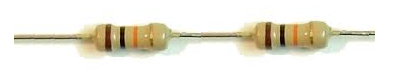﻿ How to Divide Voltage in Half with Resistors ﻿# How to Divide Voltage in Half with ResistorsIn this project, we will show how you can divide the voltage from a voltage source so that you can obtain half the voltage.

Say, a circuit is supplied with 5V across its main power line but a certain component of the circuit such as a chip only needs 2.5V.

We will show in this project how you can divide this voltage in half simply by using 2 resistors.

This circuit is very simple, yet very powerful because it shows how voltage can be reduced simply by using 2 resistors.

When we place 2 resistors of the same value in series, voltage will divide equally among them. Voltage is directly proportional to resistance, according to ohm's law by the following formula, Voltage= Current * Resistance (V= IR). So if 2 resistors have the same resistance value, an equal amount of voltage will drop across the two of them. So if we have a voltage of 5V supplying a voltage divider circuit and we place 2 resistors of equal value in series, each resistor will drop 2.5V across its terminals.

We will explain this in more detail below.

Components Needed

• 2 10KΩ resistors
• Jumper wires

You do not need to use 10KΩ resistors. You can really use any resistor value as long as the 2 resistors are the same value. The circuit will work the same.

### Voltage Divider Circuit for Half the Voltage

The voltage divider circuit which allows us to obtain half the voltage from the voltage supply is shown below.The breadboard schematic of the above circuit is shown below.As you can see, the circuit is basic.

Voltage divides across it according to the formula below.For voltage division equally in half, equal resistor values are used in a voltage divider circuit.

But you may not want half the voltage. And you don't have to. You can obtain any voltage amount from a power supply that is lower than the power supply voltage. Maybe you want 3.5V from a 5V voltage line. Or maybe you may want 2V. In this case, you simply calculate which R2 resistor value you would need in order to obtain that voltage.

If you wanted to know which resistor, R2, you should select to produce a certain desired output voltage, you can rerrange the formula above to obtain the formula seen below.With this formula, you can calculate which resistor value you would need to create any voltage output. You just choose the R1 resistor value you would like to use and then plug in the supply voltage along with the desired voltage wanted from this supply voltage into the formula.

With this formula, you could calculate the resistor needed to obtain any voltage you want.

Again, these voltage divider circuits are very basic, yet extremely powerful because they allow you to reduce voltage from a power supply to any voltage amount desired, simply by mathematically choosing the correct resistor values. So, for example, if you wanted 3V from a 5V power source, we could use a 10KΩ for R1 and a 15KΩ resistor for R2. The value across the R2 is what is output across the jumper wire. So 2V falls across R1 and 3V across R2. When we place a jumper wire in between the 2 resistors, it is the voltage that is dropped across R2 that is output on the jumper wire. So in this example, it would be 3V. So, remember in a voltage divider circuit composed of 2 resistors with a jumper wire placed in between, the voltage that will output across the jumper wire is the voltage that falls across the R2 resistor, not R1.

To use our resource to calculate the right R2 resistor value to obtain a certain desired voltage from a power supply, see Voltage Divider Resistor Calculator.

Mastering this type of circuit is very useful as a circuit designer/engineer. Because reducing voltage by using resistors is the absolute cheapest way of voltage reduction. And voltage reduction is practically used in any type of intermediately-advanced circuit. You will need to reduce voltage from the power supply to feed into components such as chips and sensors. So it is extremely common and needed for circuit design. Other ways of reducing voltage are much more expensive and burdensome such as using fixed or adjustable voltage regulators, which are far more expensive than resistors. Thus, dividing voltage by resistors is very useful.

Related Resources